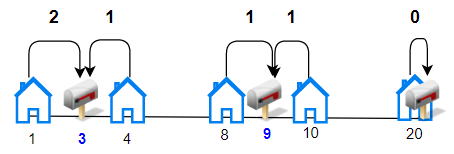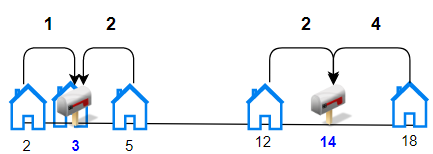# 安排邮筒

## 1478. 安排邮筒 (Hard)```输入：houses = [1,4,8,10,20], k = 3

``````输入：houses = [2,3,5,12,18], k = 2

```

```输入：houses = [7,4,6,1], k = 1

```

```输入：houses = [3,6,14,10], k = 4

```

• `n == houses.length`
• `1 <= n <= 100`
• `1 <= houses[i] <= 10^4`
• `1 <= k <= n`
• 数组 `houses` 中的整数互不相同。

### 相关话题

[数组] [数学] [动态规划] [排序]